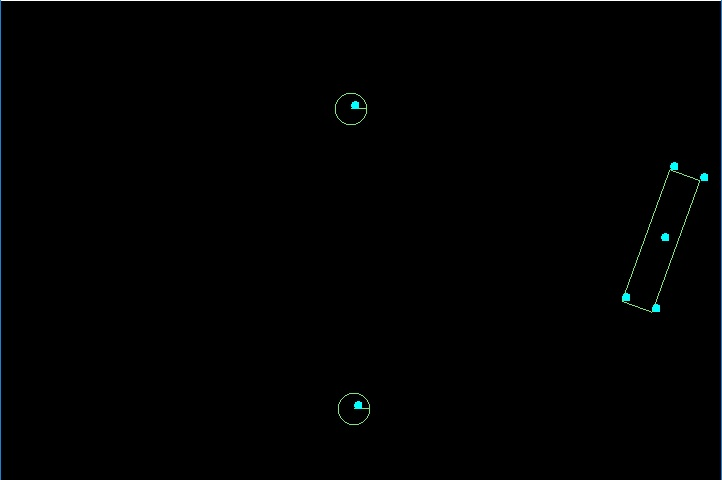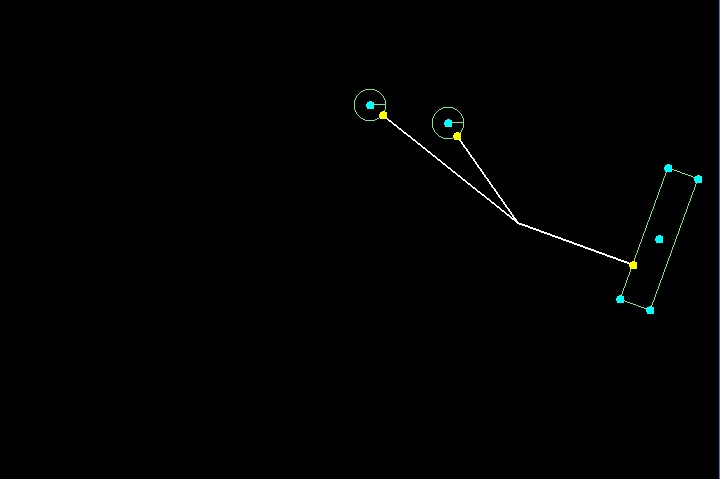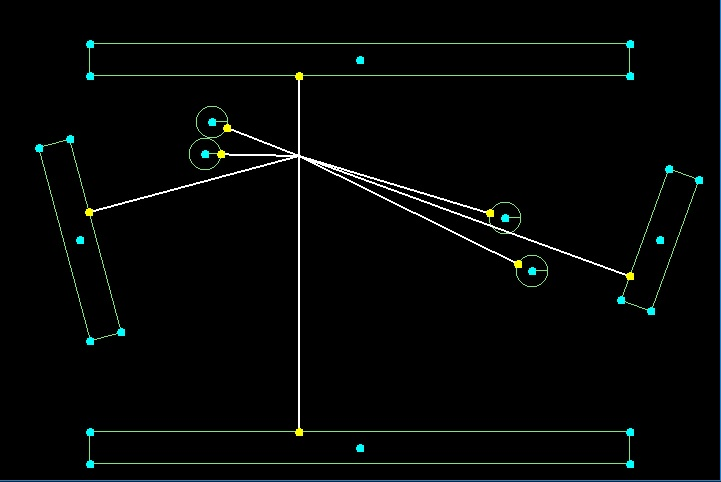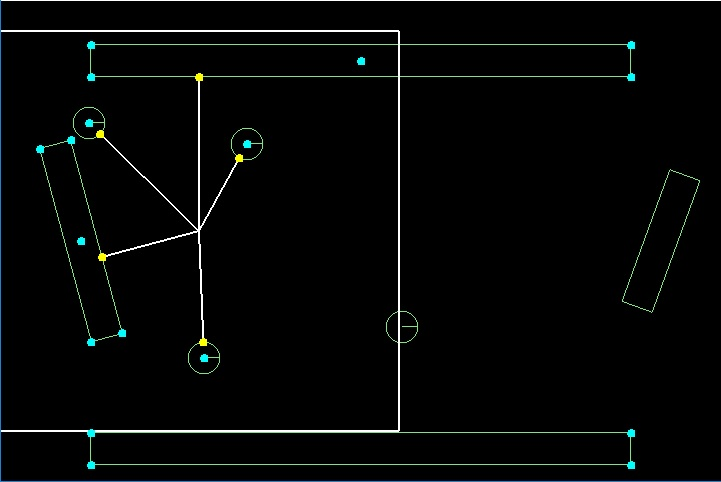# Calculate closest point of box2d body in libgdx

In this tutorial we will calculate closest point of a contact of a body from another point in box2d in libgdx. In last two tutorials, one using BoundingBox and other using Raycast we queried box2d to get list of nearby bodies. This was used to imitate explosion effect in libgdx. In bounding box we got a list of bodies in a rectangle rather than a circle. In raycast method, accuracy depends on the number of rays we generate. But in our case, we are dealing with only simple shapes like polygon and circle. So to get if the selected bodies in Bounding Box are in collision radius, we can use one more way to check it.

#### Get fixture vertices of a body in Box2d in libgdx

For circle shape, it is easy to get the closest point of contact of circle from center of explosion. We can get the distance from the center of circle to center of explosion and deduct the circle radius. It would give a point on its circumference which is the nearest point. For polygon shape, we would have to apply some more mathematics. In polygon shape we would have to first get the vertices of polygon in box2d. In box2d, we can get list of fixtures attached to a body. From fixture we can get the shape details. In shape object, the vertices information is relative to body position which we would have to translate to world coordinates.Body center and Fixture vertices highlighted in Cyan
```public static Array<Vector2> GetBodyVertices(Body body)
{
Array<Vector2> vertices=new Array<Vector2>();
for(int i=0;i<body.getFixtureList().size;i++){
//Get Fixture
Fixture f=body.getFixtureList().get(i);
//Check fixture shape
if(f.getType()== Shape.Type.Polygon){
//Cast the shape to polygon shape
PolygonShape ps=(PolygonShape)f.getShape();
for(int j=0;j<ps.getVertexCount();j++){
Vector2 v=new Vector2();
//Get vertex in v variable
ps.getVertex(j,v);
//Add body position to vertex to get world position
v.add(body.getPosition());
//Rotate around body center to add body rotation to the vertex
v.rotateAround(body.getPosition(),body.getAngle()* MathUtils.radiansToDegrees);
vertices.add(v);
}
}
}
return vertices;
}```

In the above code we are getting vertices only for Polygon shapes. First we check if the fixture is a polygon. Then we cast the shape to PolygonShape class. Using getVertexCount we get count of vertices. We add body position to the vertex to get its world position. Then rotate it around body center to add rotation, if any, to fixture vertices.

#### Calculate closest point on a line segment from another point

Now we have the vertices of polygon shapes attached of our bodies in box2d. The closest point on the body can be one of the vertex or on a line segment between these vertices. We will now add a method where we would pass two adjacent vertices of a fixture and the point from where we have to check the distance of this line segment.Line segments from point of click on screen to nearest points on the bodies.
```private static Vector2 GetClosestPointInLine(Vector2 point,Vector2 linePoint1,Vector2 linePoint2){
Vector2 closest=new Vector2();
Vector2 bVec1=new Vector2();
Vector2 bVec2=new Vector2();
bVec1.set(linePoint2).sub(linePoint1); //LINE FROM POINT 1 to 2
bVec2.set(point).sub(linePoint1); //LINE FROM VERTEX TO POINT 1
float av=bVec1.x*bVec1.x+bVec1.y*bVec1.y;
float bv=bVec2.x*bVec1.x+bVec2.y*bVec1.y;
float t=bv/av;
//IF POINT LIES OUTSIDE THE LINE SEGMENT, THEN WE FIX IT TO ONE OF THE END POINTS
if(t<0) t=0;
if(t>1) t=1f;
//Closest point = point 1 + line from point 1 to 2 * t
closest.set(linePoint1).add(bVec1.x*t,bVec1.y*t);
return closest;

}```

In the above snippet we are passing the outside point and line segment points as parameter. Then we are doing some mathematics to get closest point on the line. As this is a line segment, we are checking if closest point is inside the segment or not. If not, then closest point is one of the end points of the line segment.

#### Get minimum distance of Body from Point

We are now able to get vertices of a body in box2d in libgdx, albeit of a simple polygon shape. We can also calculate the closest point of a line from another point in space. Now we use these two methods together to get minimum distance of a body from point. As we mentioned above, this method is only for simple polygon and circle shapes. For more complex shapes, maybe we would add a solution in future.Nearest points of bodies from the click point
```private static float  BOX_TO_WORLD=100f;
private  static float   WORLD_TO_BOX =0.01f;
public static float ConvertToBoxCoordinate(float v){
return v*WORLD_TO_BOX;
}

public static float ConvertToWorldCoordinate(float v){
return v*BOX_TO_WORLD;
}
//THIS HANDLES ONLY POLYGON SHAPES
//AND A CIRCLE SHAPE
public static Vector2 GetClosestPoint(Body b, Vector2 startPoint){
Vector2 closestPoint=new Vector2();
//Convert to Box Coordinates
float sx=ConvertToBoxCoordinate(startPoint.x);
float sy=ConvertToBoxCoordinate(startPoint.y);
Vector2 boxPoint=new Vector2(sx,sy);
float minDis=Float.MAX_VALUE;
Array<Vector2> vs=GetBodyVertices(b);
boolean supportedShape=false;
for(int i=0;i<vs.size;i++){
supportedShape=true;
Vector2 closest=GetClosestPointInLine(boxPoint,vs.get(i),vs.get((i+1)%vs.size));
float d=closest.dst2(boxPoint);
//Loop through edges to find the edge closest to the point
if(d<minDis) {
minDis=d;
closestPoint.set(closest);
}
}
if(b.getFixtureList().size>0 && b.getFixtureList().get(0).getType()== Shape.Type.Circle){
supportedShape=true;
CircleShape cs=(CircleShape) b.getFixtureList().get(0).getShape();
float distance=boxPoint.dst(b.getPosition()); //GET DISTANCE OF BODY CENTER FROM START POINT
float circumferenceDistance=distance-cs.getRadius();  //DEDUCT RADIUS TO GET POINT ON CIRCUMFERENCE
closestPoint.set(b.getPosition()).sub(boxPoint).scl(circumferenceDistance/distance).add(boxPoint);
}
if(supportedShape)
return closestPoint;
return  null;
}```

In the above code we are passing Body and the point as parameters. First we are converting point to box coordinates as in our application box and world coordinates have a ratio of 1:100. Then for a polygon shape, we are getting the vertices for a body. We loop through them and get the closest point by comparing the distance for all the edges of the fixture of the body. For circle shape, it is much simpler. We get the distance between point and center of circle body and deduct the radius from it.

#### Using Bounding Box QueryAABB and closest point together

Till now we are able to get vertices of bodies of polygon shape, closest point on a line from another point and closest point on a body from another point. We can loop through the bodies to check if they are in explosion radius. But it would be inadvisable to loop through all the bodies in world, to calculate this list. In last tutorials we made use of bounding box and raycast to calculate this list. So we can use bounding box to narrow down the number of bodies. In that list we can calculate the nearest point of body and check if it falls inside the explosion radius. For more details on QueryAABB in box2d in libgdx, you can check out the tutorial here.Looping through the bodies queried in bounding box to check if they fall inside the explosion radius
```QueryCallback bbCallback; //callback for queryaabb
float blastRadius;  //explosion radius
Array<Body> boundingboxBodies; //array to store bodies returned in queryaabb
Array<Vector2> closestPoints; //array to store closest points of the bodies from click point
Vector2 touchUpPoint; //mouse click point
World  world ;
public void Init(){
...
blastRadius=200 ;
boundingboxBodies =new Array<Body>();
touchUpPoint=new Vector2();
closestPoints=new Array<Vector2>();
bbCallback=new QueryCallback() {
@Override
public boolean reportFixture(Fixture fixture) {
boundingboxBodies.add(fixture.getBody());
//To keep on checking other fixtures, return true
return true;
}
};
...
}

@Override
public boolean touchUp(float screenX, float screenY, int pointer, int button) {
touchUpPoint.set(screenX,screenY); //store click point
CheckBoundingBox(); //query bodies for explosion
return false;
}

private void CheckBoundingBox(){
boundingboxBodies.clear();
float x=ConvertToBoxCoordinate(touchUpPoint.x) ;
float y=ConvertToBoxCoordinate(touchUpPoint.y) ;
float boxBlastRadius=ConvertToBoxCoordinate(blastRadius) ;
world.QueryAABB(bbCallback,x-boxBlastRadius,y-boxBlastRadius,
x+boxBlastRadius,y+boxBlastRadius);
CalculateClosestPoints();

}

private void CalculateClosestPoints(){
closestPoints.clear();
for(int i=0;i<boundingboxBodies.size;i++){

Vector2 cp=GetClosestPoint(boundingboxBodies.get(i),touchUpPoint);
if(cp!=null) {
Vector2 cpw=new Vector2(ConvertToWorldCoordinate(cp.x), ConvertToWorldCoordinate(cp.y));
//CHECK DISTANCE
float dst2=cpw.dst2(touchUpPoint);
if(dst2<=(blastRadius*blastRadius)) {
closestPoints.add(cpw);
}
}
}

}```

#### Full Libgdx Source Code

The above solution may not work for many cases, but it is useful for case when bodies are of simple shapes (polygon and circle). In some cases raycast may make sense where one body may obstruct explosion of other body. Though for us this was not the scenario we needed to handle, so it works fine for us.

Here is link to github code repository of libgdx examples which has this snippet along with other examples covered in previous tutorials.

Tagged with: , ,